What Steps should one take while doing Data Preprocessing?

What is Data Preprocessing?

Data preprocessing is a data mining technique that involves transforming raw data into an understandable format. Real-world data is often incomplete, inconsistent, and/or lacking in certain behaviors or trends, and is likely to contain many errors. Data preprocessing is a proven method of resolving such issues.

Why we use Data Preprocessing?

In Real world data are generally incomplete: lacking attribute values, lacking certain attributes of interest, or containing only aggregate data. Noisy: containing errors or outliers. Inconsistent: containing discrepancies in codes or names.

Steps in Data Preprocessing

Step 1: Import the libraries

Step 2: Import the data-set

Step 3: Check out the missing values

Step 4: See the Categorical Values

Step 5: Splitting the data-set into Training and Test Set

Step 6: Feature Scaling

So, without wasting further time let’s get started!!!

“ New beginnings often starts with painful endings. Be thankful for those closed doors and detours, they are guiding you to a better place”

Step 1 : Import the Libraries

This is how we import libraries in Python using import keyword and this is the most popular libraries which any Data Scientist used. (I used- Jupyter Notebook)

• NumPy is the fundamental package for scientific computing with Python. It contains among other things:

1. A powerful N-dimensional array object

2. Sophisticated (broadcasting) functions

3. Tools for integrating C/C++ and FORTRAN code

4. Useful linear algebra, Fourier transform, and random number capabilities

• Pandas is for data manipulation and analysis. Pandas is an open source, BSD-licensed library providing high-performance, easy-to-use data structures and data analysis tools for the Python programming language. Pandas is a NumFOCUS sponsored project. This will help ensure the success of development of pandas as a world-class open-source project and makes it possible to donate to the project.
• Matplotlib is a Python 2D plotting library which produces publication quality figures in a variety of hardcopy formats and interactive environments across platforms. Matplotlib can be used in Python scripts, the Python and IPython shells, the Jupyter notebook, web application servers, and four graphical user interface toolkits.
• Seaborn is a Python data visualization library based on matplotlib. It provides a high-level interface for drawing attractive and informative statistical graphics.
• Warning messages are typically issued in situations where it is useful to alert the user of some condition in a program, where that condition (normally) doesn’t warrant raising an exception and terminating the program. For example, one might want to issue a warning when a program uses an obsolete module.

Step 2 : Import the Data-set

By using Pandas we import our data-set and the file I used here is .csv file [Note: It’s not necessarily every-time you deal with CSV file, sometimes you deal with Html or Xlsx(Excel file) ]. However, to access and to use fastly we use CSV files because of their light weights. After importing the dataset, you can see we use head function ( This function returns the first n rows for the object based on position. It is useful for quickly testing if your object has the right type of data in it. By default it returns 5 rows. )

Step 3 : Check out the Missing Values

The concept of missing values is important to understand in order to successfully manage data. If the missing values are not handled properly by the researcher, then he/she may end up drawing an inaccurate inference about the data. Due to improper handling, the result obtained by the researcher will differ from ones where the missing values are present.

Yes, you have seen it write this number 3720 is your missing values in each column.

Maybe or May not you have read somewhere, see 7 techniques to deal with Missing Values or 5 ways to deal with Missing Values. But, I will discuss with you only those two ways which are the most prominent.

Two ways to handle Missing Values

1. This method commonly used to handle null values. Here, we either delete a particular row if it has a null value for a particular feature and a particular column if it has more than 75% of missing values. This method is advised only when there are enough samples in the dataset. One has to make sure that after we have deleted the data, there is no addition of bias. Removing the data will lead to the loss of information which will not give the expected results while predicting the output.

2. This strategy can be applied on a feature which has numeric data like the year column or Home team goal column. We can calculate the mean, median or mode of the feature and replace it with the missing values. This is an approximation which can add variance to the data set. But the loss of the data can be negated by this method which yields better results compared to removal of rows and columns. Replacing with the above three approximations are a statistical approach of handling the missing values. This method is also called leaking the data while training. Another way is to approximate it with the deviation of neighboring values. This works better if the data is linear.

Above strategy is good for numeric data. But what happens when Categorical data has missing values?

Step 4 : See the Categorical Values

Let’s see how to deal with Categorical Data now . . . !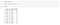See the Categorical Data in Country

Since, machine learning models are based on Mathematical equations and you can intuitively understand that it would cause some problem if we can keep the Categorical data in the equations because we would only want numbers in the equations.

So, we need to encode the Categorical Variable…..

Like in our dataset Country column will cause a problem, so will convert into numerical values. To convert Categorical variable into Numerical data we can use LabelEncoder() class from preprocessing library.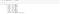See the Categorical data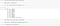Use LabelEncoder class to convert Categorical data into numerical one

label_encoder is an object which is I use and help us in transferring Categorical data into Numerical data. Next, I fitted this label_encoder object to the first column of our matrix X and all this return the first column country of the matrix X encoded.

But there is a problem in it, the problem is still the same, machine learning models are based on equations and that’s good that we replaced the text by numbers so that we can include the numbers in the equations.

However, since 1>0 and 2>1(See the above data-set), the equations in the model will think that Spain has a higher value than Germany and France, and Germany has a higher value than France.

Actually, this is not the case, these are actually three Categories and there is no relational order between the three. So, we have to prevent this, we’re going to use what are Dummy Variables.

What are Dummy Variables?

Dummy Variables is one that takes the value 0 or 1 to indicate the absence or presence of some categorical effect that may be expected to shift the outcome.

Instead of having one column here above, we are going to have three columns.

Number of Columns = Number of Categories

In our case, we going to have 3 columns.

// To create dummy variable we are going to use OneHotEncoder Class from sklearn.preprocessing or you can use pandas get dummies method.

In the above code, I already explain how to use OneHotEnCoder Class, now I will show you with pandas how to use get_dummies( ) for creating Dummy Variables.

Sometimes, we use KNN Imputation(for Categorical variables): In this method of imputation, the missing values of an attribute are imputed using the given number of attributes that are most similar to the attribute whose values are missing. The similarity of the two attributes is determined using a distance function.

Step 5 : Splitting the data-set into Training and Test Set

In any Machine Learning model is that we’re going to split data-set into two separate sets

1. Training Set

2. Test Set

Why do we need splitting?

Well here it’s your algorithm model that is going to learn from your data to make predictions. Generally, we split the data-set into 70:30 ratio or 80:20 what does it mean, 70 percent data take in train and 30 percent data take in the test. However, this Splitting can be varied according to the data-set shape and size.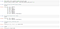Splitting the Data-set into two set — Train and Test Set

X_train is the training part of the matrix of features.

X_test is the test part of the matrix of features.

y_train is the training part of the dependent variable that is associated to X_train here.

y_test is the test part of the dependent variable that is associated to X_train here.

Step 6 : Feature Scaling

What is Feature Scaling?

Feature scaling is the method to limit the range of variables so that they can be compared on common grounds.

Suppose we have this data-set

See the Age and Salary column. You can easily notice the Salary and Age variable don’t have the same scale and this will cause some issue in your machine learning model.

Because most of the Machine Learning models are based on Euclidean Distance.

Let’s say we take two values from Age and Salary column

Age- 40 and 27

Salary- 72000 and 48000

One can easily compute and see that Salary column will be dominated in Euclidean Distance. And we don’t want this thing.

So, there are several ways of scaling your data. Reference

The picture was taken from Wikipedia feature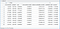After Feature Scaling all values comes into the same scale

Note: Sometimes machine models are not based on Euclidean distances, we will still need to do features scaling because the algorithm will converge much faster. That will be the case for Decision Tree which is not based on ED but, if we do not do feature scaling then they will run a very long time.

So that’s our end with Data Preprocessing phase and I hope you like this Article . . . !! Please note I have not included outliers topic in it and taken very simple data-set to make you understand everything. Please feel free to comment and give some applaud on it. If you have any problem or query in any topic related to Data Science then do let me know in the comment Section. We’ll share more articles soon on TheMenYouWantToBe&Co. Article section as well as on Medium.

Subscribe now ❤ and Give some love too!

Written by

Mohit Sharma

Incedge offers interactive R, Python, Machine Learning and Deep Learning blogs. Our blogs will help you get ahead in field of Data Science. Practical Exposure. Come along with us to make a world a better place to live.

Welcome to a place where words matter. On Medium, smart voices and original ideas take center stage - with no ads in sight. Watch
Follow all the topics you care about, and we’ll deliver the best stories for you to your homepage and inbox. Explore
Get unlimited access to the best stories on Medium — and support writers while you’re at it. Just \$5/month. Upgrade